Closescroll

# Math Function Optimization with Python

Very often it is necessary to calculate some practical examples for optimizing the parameters of a particular model in economics, technology, and project management, etc. The most common optimization option is finding a minimum for a function of one variable. How to solve such a problem without spending much time and using some simple programming language, such as Python? Let's look at this problem.

## What is a function?

Function (mapping, operator, transformation) in mathematics, is the correspondence between the elements of two sets, established by such a rule that each element of the first set corresponds to one and only one element of the second set.

The mathematical concept of a function expresses an intuitive idea of how one quantity completely determines the value of another quantity. For example, the value of the variable x uniquely determines the value of the expression x2.

It is said that on the set X there is a function (map, operation, operator) f with values from the set Y if each element x from the set X is assigned, according to the rule f, some element y from the set Y.

It is also said that the function f maps the set X to the set Y. The function is also denoted by the notation y = f (x).

## What is optimization?

Optimization in mathematics, computer science, and operations research, the problem of finding the extremum (minimum or maximum) of a target function in a certain area of a finite-dimensional vector space limited by a set of linear and/or nonlinear equalities and/or inequalities.

In the design process, the task is usually to determine the best, in a sense, structure, or parameter values of objects. Such a task is called optimization. If optimization is associated with the calculation of optimal parameter values for a given object structure, then it is called parametric optimization.

Optimization allows you to find the best combinations of parameters, for example, the number of workers to perform a specific task, the best route for vehicles with fuel economy, the ratio of weight and structural strength, etc.

The most common optimization methods are implemented in the scipy.optimize library. Optimization methods are divided into gradient and gradientless. For gradient optimization methods, it is necessary to analytically set the derivative function for each variable. Gradient methods have a higher convergence rate. The non-gradient methods are slower, but allow you to calculate more complex functions, without a complicated manual derivation procedure.

The most common methods for optimizing the function of one variable are the uniform search method, the dichotomy method, the golden ratio method, the fastest descent method (gradient).

## How to optimize function in Python

Let's see how to solve the optimization problem quickly and efficiently using Python, the scipy library, and the Google Colab cloud system. Open Google Colab and create a new project. We define a function that we will minimize: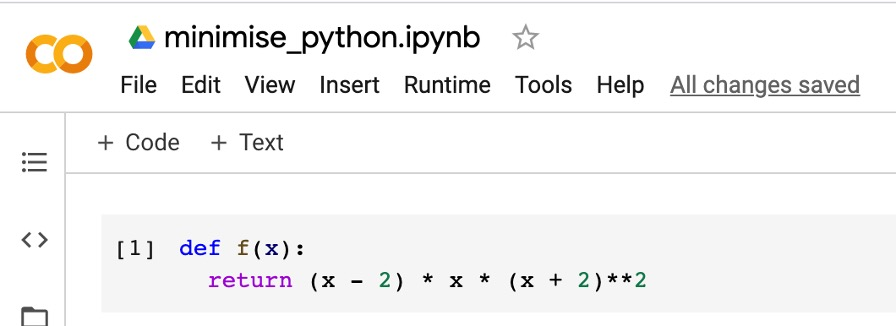Let's try to give the input the value of the argument and check how the function is calculated: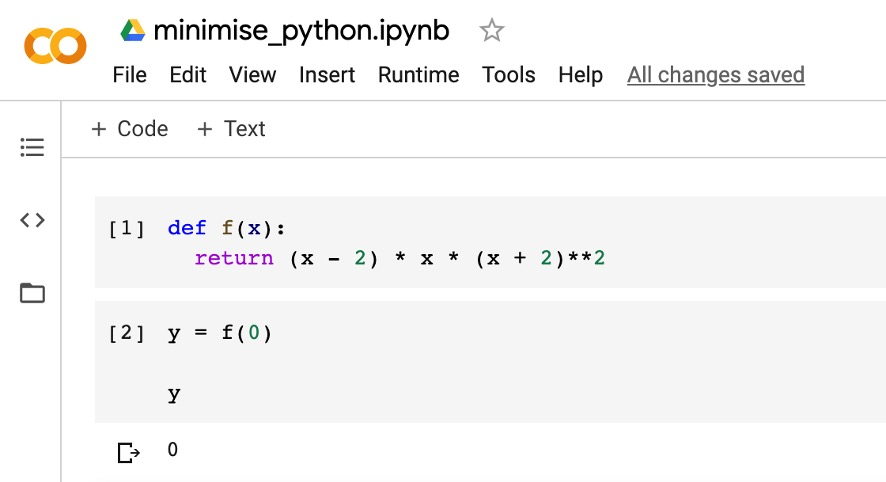Let’s connect the scipy library: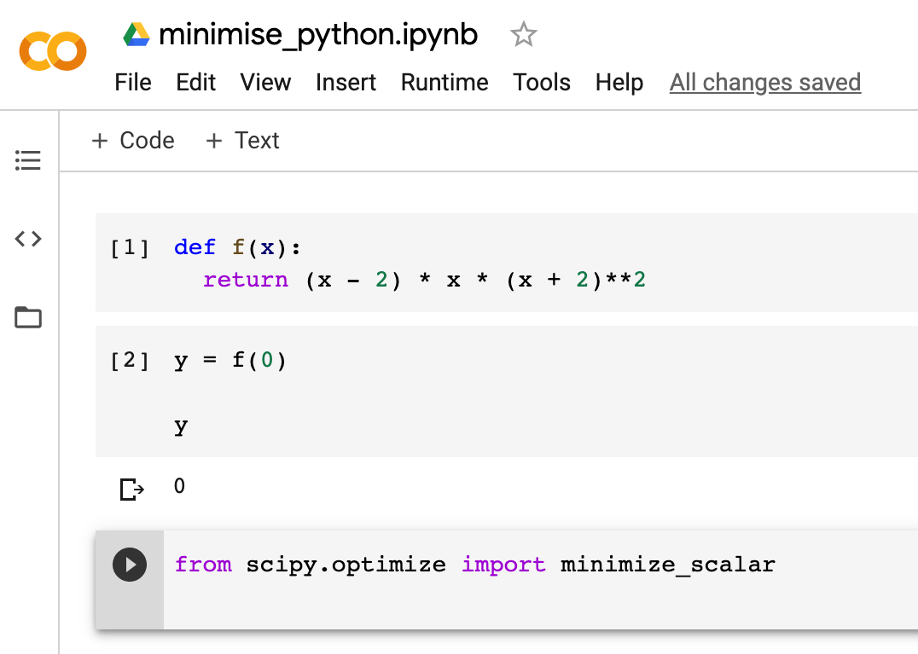And run the optimization function to find the minimum. We use the minimize_scalar () function

See the documentation for this function here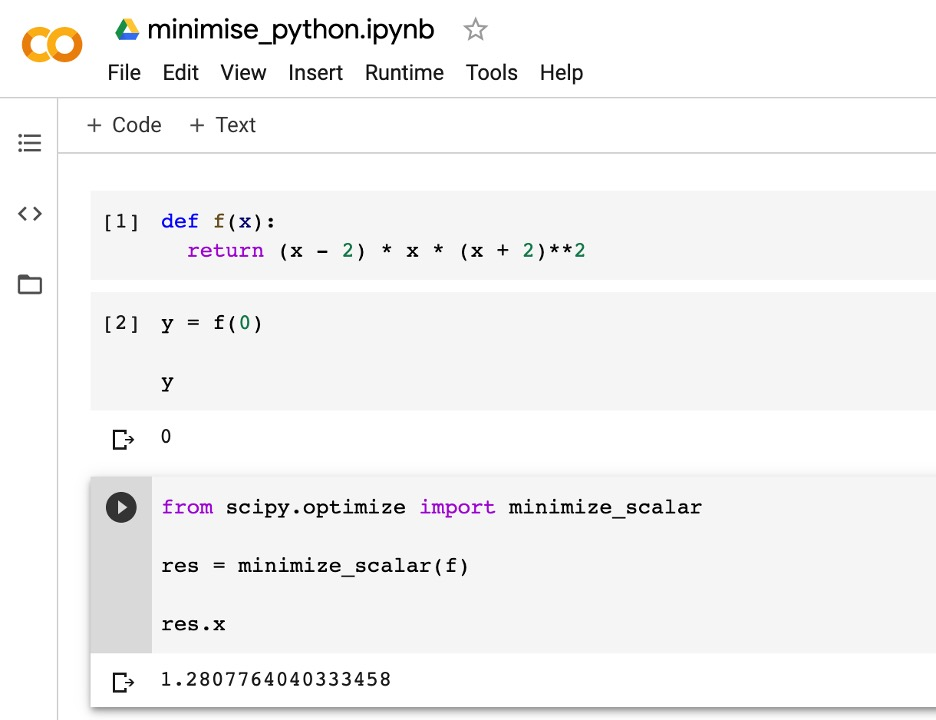Now substitute this value into the function and see what happens: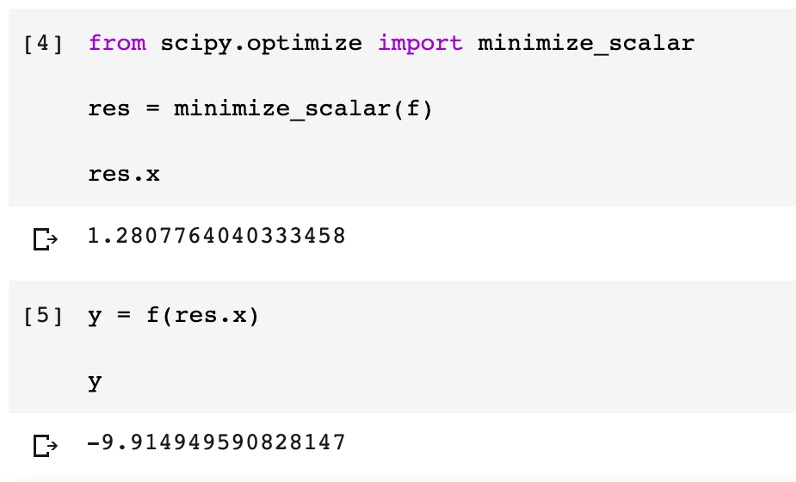Now let's see how it looks on the plot: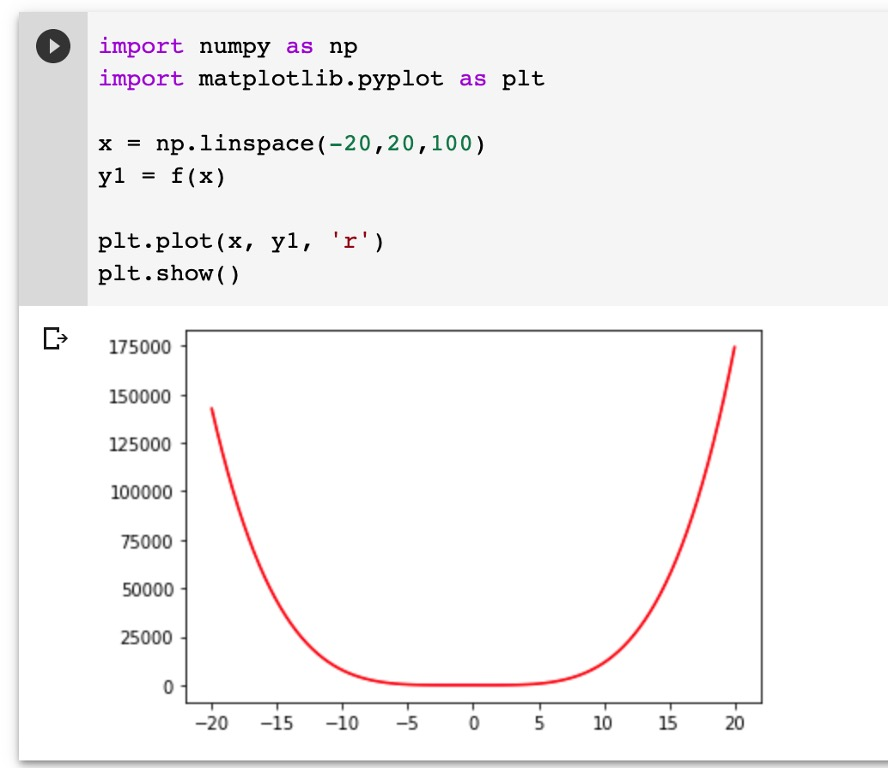And now we will display the same graph closer to the minimum definition area: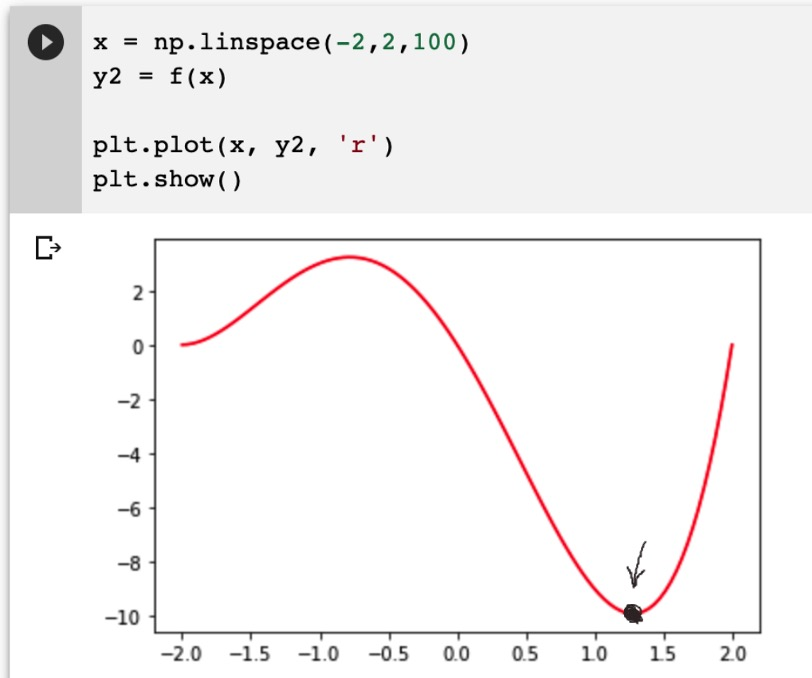So we got the minimum point of the function, x = 1.2807764040333458, y = -9.914949590828147, which is very clearly visible on the graph.

Also, in the scipy.optimize.minimize_scalar function, you can use optimization methods such as ‘Brent’, ‘Bounded’, Golden’ and write your own custom optimization method.

And if you look more broadly at the possibilities of optimizing mathematical functions in the scilab library, then you can apply:

• Conditional and unconditional minimization of scalar functions of several variables (minim) using various algorithms (Nelder-Mead simplex, BFGS, conjugate Newton gradients, COBYLA, and SLSQP).
• Global optimization (ex: basinhopping, diff_evolution).
• Minimization of residuals of least squares (least_squares) and algorithms for fitting curves to non-linear least squares (curve_fit).
• Minimization of scalar functions of one variable (minim_scalar) and search for roots (root_scalar).
• Multidimensional solvers of the system of equations (root) using various algorithms (hybrid Powell, Levenberg-Marquardt, or large-scale methods, such as Newton-Krylov).

## Conclusion

In conclusion, we note that in modern cloud systems, such as Google Colab, all the necessary libraries for solving optimization problems are already installed, plus there are libraries for drawing graphs. That is, in practice, you can take your target function, for example, in calculating the economic parameters of your enterprise and develop optimal characteristics. For example, you could determine the number of materials in the warehouse needed to produce the right amount of products. In Python, you can write two lines of code and find the optimal parameters.

Our specialists from Svitla Systems will help you specify the necessary requirements for solving such problems. We possess the necessary knowledge and mathematical training to solve large-scale problems. Optimally working with customers, the company delivers the best solution at the right time with cost savings on the project budget, which is very important for modern conditions in 2020.

by Svitla Team
July 07, 2020